TRIGONOMETRY FOR STATICS

PART 3:

For 0o and 90o: These functions are limiting values that can be observed from the drawing. As side y approaches 0 (zero), the x approaches r.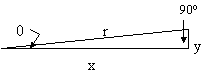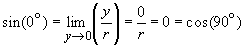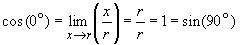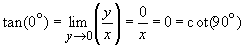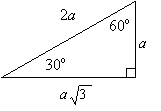For 30o and 60o: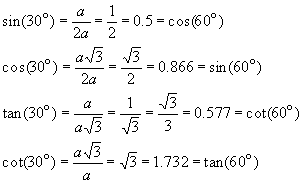For 45o: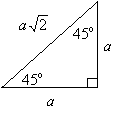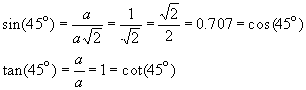Basic identities: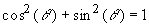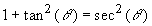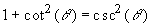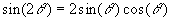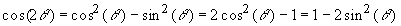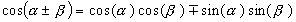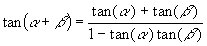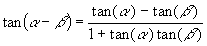Half-angles: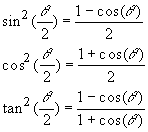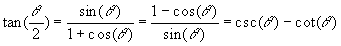Identities in terms of tan (q/2):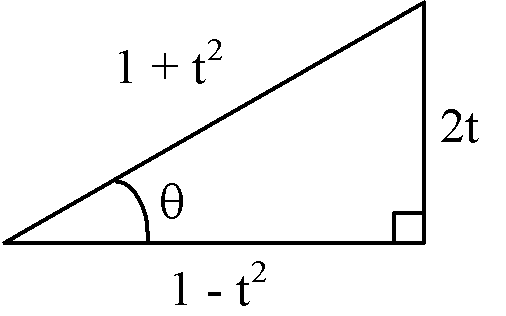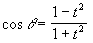where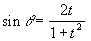Sine function: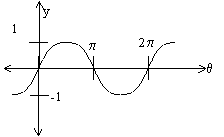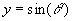The sine function is an odd function since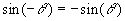Cosine function: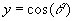The cosine function is an even function since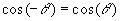Tangent function: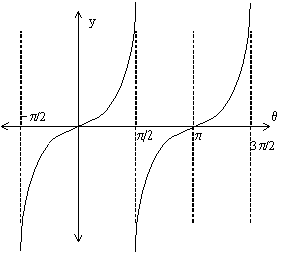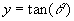The tangent function is an odd function since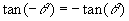Note that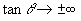as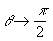As a close approximation, when an angle(expressed in radians) is very small, we may use the following approximations

• replace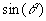with• replace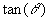with• replace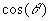with unity (i.e.,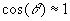)

GO BACK TO PART 2